# Algebra 1

Teacher: Canaa
Customers Who Have Viewed This Course: 2084
\$179.00

#### 1 Welcome to Ms. Lee's Algebra Class 08:54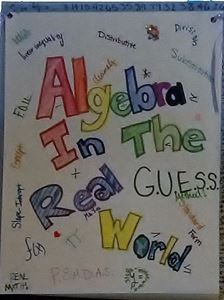I am excited about spending the next several months with you in the wonderful world of algebra. I have provided you a sneak peek at a few of the innovative and engaging lessons that help you grasp and understand the fundamental principles of algebra. In this “algebra trailer” you can preview what you are going to learn throughout the course. I look forward to working with you! See you soon!

#### 2 Lesson 1: Classification of Sets of Real Numbers 21:03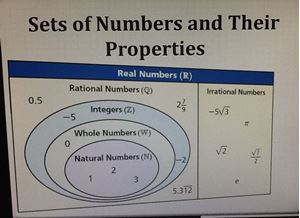In this lesson, students learn the system that governs and classifies. Numbers are not random, but follow rules and follow into six subsets of numbers in the real number system. Students will learn the six subsets of numbers. When given numbers, students will be able to determine which categories the numbers belong.

#### 4 Lesson 2: Operations and Properties of Real Numbers 14:10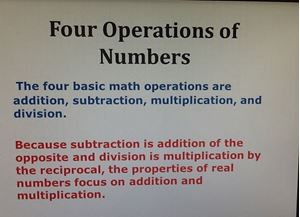In this lesson, students learn how the four basic operations of numbers (addition, subtraction, multiplication and division) are applied to the properties of numbers. These properties are essential as we learn to simplify expressions and solve equations. In addition, vocabulary is necessary to a successful learning experience.

#### 6 Lesson 3: Arithmetic Properties of Integers 25:53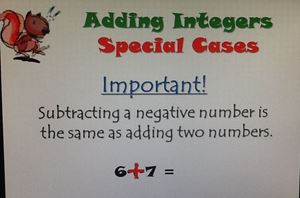In this lesson, students learn the rules that govern integers. In lesson 2, we learned what integers are. In this lesson, we apply the rules of integers for addition, subtraction, multiplication and division. It is important to master these rules because we need them when we start solving linear equations and inequalities.

Integers

#### 8 Lesson 4: Arithmetic Properties Set Notation 19:44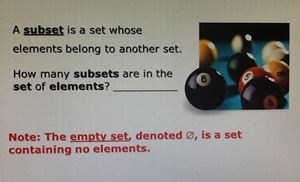In this lesson, we learn how to represent a set of number using mathematical language. There are a lot of symbols in math because if we did not use symbols and short hand, we would more time writing than doing math. The challenging part is to identify the symbol and know it means. I am confident it will be a piece of cake!

Set Notation

#### 10 Lesson 5: The Closure Property 12:55

In this lesson, we learn that when you add two real numbers, we get a real number every time! We know this is always true. However, every operation does not produce a number that belongs to its category. We will look at the four operations and determine which operations are closed and which operations are not closed.

#### 12 Lesson 6: True and False Assertions 13:11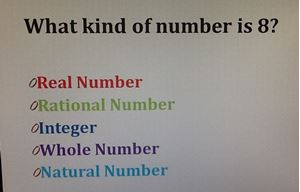Is there enough proof for the statement to be TRUE? If not, we have to apply the properties and rules we have learned apply them.  Our goal is to prove the validity of the statement. This lesson helps us think logically and give reasonable evidence for our answers.

#### 14 Lesson 1: Can You Tell Me the Order? 17:40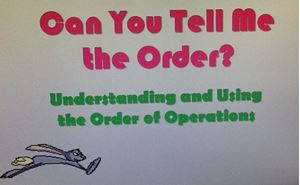In module 1, we discussed the laws and properties that govern the order and the ranks of sets of numbers in the real number system. In this lesson 1, we are going to apply the properties of numbers. In this lesson is called, “Can You Tell Me the Order?” because students apply the rules and the operations of numbers to determine the correct answer. Students discover the order of the operations matter when working out the problem.  You may find a four-function is helpful for the independent practice.

#### 16 Lesson 2: Understanding and Using Operations: Opposites and Reciprocals 10:56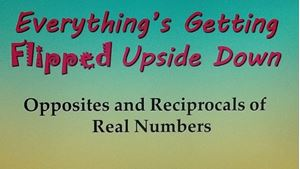In Module 1, we learned about the additive inverse and the multiplicative inverse of a number. It will be helpful to refer back to the properties of numbers in module 1 because these properties are used in module 2. Students use these properties when talking about opposites and reciprocals. We discover that the additive inverse is called the opposite of a numbers; the multiplicative inverse is called the reciprocal of a number. These four terms are the essence of this lesson. However, the mathematics behind these words is a piece of cake!

#### 18 Lesson 3: What's the Root of the Problem? 18:00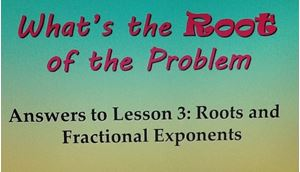In lesson 3, students learn about properties of square roots and cube roots. This concept helps us better understand fractional powers. Don’t worry! Fractional powers are not as frightening as they may sound. We find the product of the root and whole number powers to find the answer. I have provided a list of square roots and cube roots that is very helpful as we proceed through this lesson.

#### 20 Lesson 4: Laws of Exponents 19:37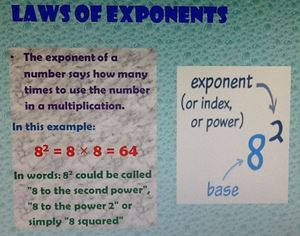In this lesson, we investigate at the rules and properties that govern exponents. An exponent is short hand for multiplication. It is very important for students to know and be able to apply all the laws of exponents. We find that there is more than one law that is applied in a single problem. We also investigate the rules for exponents involving multiplication and division. In addition, we learn how the reciprocal of a number is in important when simplifying exponents.

#### 22 Lesson 5: Variables and Like Terms 17:18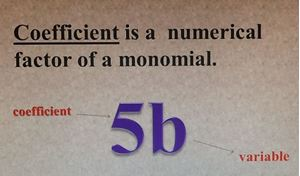In this lesson, students are introduced to the variable and the vocabulary that surrounds it. We investigate what a term is, and determine if two terms are like terms or unlike terms. This is important because this lesson is all about simplifying expressions and adding like terms together. This skill is necessary throughout the remainder of the course. It is essential for students to be proficient in simplify and combining like terms.

#### 24 Can You Accept That to be True 12:45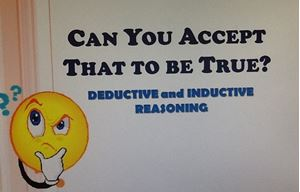In this lesson, students are introduced to two types of logical reasoning for problem solving: deductive reasoning and inductive reasoning. Mathematics primarily uses deductive reasoning for its method of finding the solution; there is one correct answer. However, when we are looking for a pattern, then we may use inductive reasoning. Students may find that inductive reasoning is mostly the logical means of reasoning in science.

#### 26 Under What Conditions is the Statement True? 06:56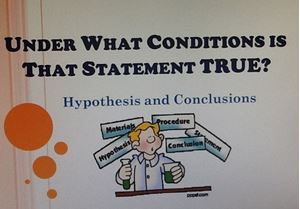In this lesson, students discover how to determine if a statement is true or false from the given information. Next, students use deductive reasoning to identify the hypothesis and conclusion of a statement. Furthermore, we are going to write a conditional statement when we are given the hypothesis and conclusion. We learn that a counterexample is an example that shows a conditional statement is false.

#### 28 Lesson 1: Simplifying Expressions 13:23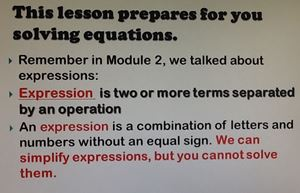This lesson is a continuation of Lesson 5 from Module 2, “Variables and Like Terms.” In lesson 1 of Module 3, we are going to simplify more complex expressions that are more commonly known as algebraic expressions. Students are to apply the Laws of Exponents, the Distributive and Property and combining like terms to successfully simplify each expression. This skill is very important when we solve linear equations in the next lesson.

#### 30 Lesson 2: Ecstatic Ellen and Equations 17:36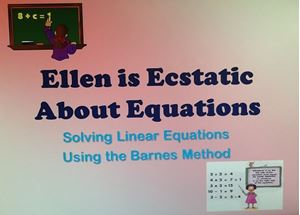In this lesson, Ecstatic Ellen is your tour guide. She is excited about solving equations! She knows a fun and an easy to way to solve the most challenging equations using the Barnes Method. Students become confident and excited about being able to solve equations! Let’s get started!

#### 32 Ecstatic Ellen and Inequalities 13:10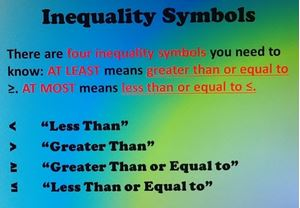In this lesson, students use the same skills needed to solve liner equation.  However, students are introduced to the four inequality symbols, determine whether circle on the number line is opened or closed, and determine whether the inequality which direction to shade on the number line.  You may find a color pencil to be helpful in this lesson.

#### 34 Lesson 4: Problem Solving 13:56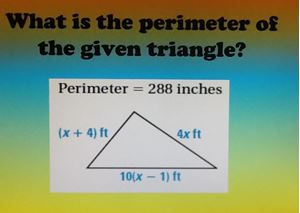There is no need to fear word problems. In this lesson, students are introduced to easy and fun way to solve word problems. It is called the GUESS Method. Students determine if the answer they calculate is reasonable. Students learn what GUESS stands for and how it use the method to confidently set up and solve word problems with ease!

#### 36 Lesson 5: Problem Solving Involving Inequalities 10:07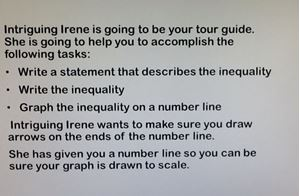In this lesson, students use the GUESS Method to solve word problems. It is important for students to make sure their answer makes sense in the context of the problem. Students have may have to round or estimate when giving their answers.

#### 38 Lesson 6: Solving Absolute Value Equations 08:39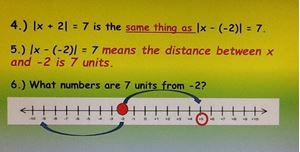In this lesson, students learn what absolute value means and it applies when solving absolute value equations. Students are expected to be able to write the two equations, solve the two equations, and describe the answers in words. Written communication is helpful in conceptualizing the answer.

#### 40 Lesson 7: Solving Absolute Value Inequalities 09:06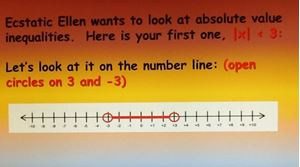In this lesson, students apply their knowledge of graphing inequalities. Students are expected to able to write the two inequalities, solve and graph the inequalities, and describe the solution in words. Written communication is helpful in conceptualizing the answer.

#### 42 Lesson 1: Graphing Linear Equations 20:43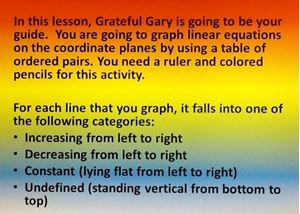In this lesson, graph paper is provided. Students need a ruler. Students are given a set of x-values they use to substitute into the equation. The y-value is the answer needed to form the ordered pairs. The ordered pairs are then plotted on the coordinate plane form the linear equation.

#### 44 Lesson 2: Graphing Linear Inequalities. 23:09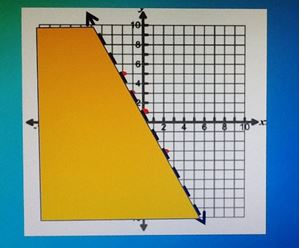In this lesson, graph paper is provided. Students need a ruler, a highlighter and colored pencils. They are some similarities in graphing equations and graphing inequalities. However, when graphing inequalities, students is to determine which area on the graph is the solution set.

#### 46 Lesson 3: Help Me Find My Equation! 19:47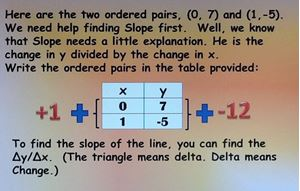In this lesson, I have written a story that involves three friends: Slope and his two friends X and Y. They are sad because they cannot find their equation. Slope, X and Y need your help to find the equation using the Point-Slope Formula. This lesson is a lot of fun!

#### 47 Help Me Find My Equation! Independent Practice 19:47

Point-Slope Formula

#### 48 Lesson 4: What’s the Slope? 06:45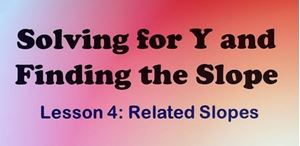In this lesson, students learn how to write a two-variable equation in slope-intercept form. Students used the Barnes Method for using x, and use it to solve the equations for y. Now that the equations are slope-intercept form, students identify the slope, y-intercept, and determine whether the line increases, decreases, constant or undefined.

#### 50 Lesson 1: Systems of Linear Equations. Part 1 19:29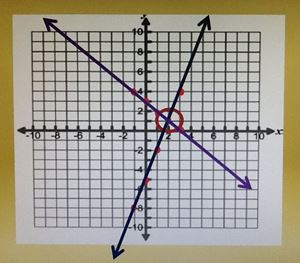There are two parts to solving systems of linear equations. In part 1 of this lesson, students solve systems of linear equations by graphing and substitution. The point of intersection is called the solution of system of linear equations when we are graphing. When using substitution, you solve the first equation for y, and substitute the FIRST into the SECOND equation. Graph paper is provided.

#### 51 Lesson 1: Systems of Linear Equations. Part 2 10:25

Elimination Method

#### 53 Lesson 2: What’s the Solution Set? 18:32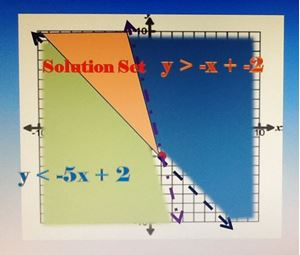In this lesson, students generate a table of values to plot on the coordinate plane. Based on the inequality symbol, students determine whether the line that is drawn is solid or dashed; students also determine whether they must shade below or above the line. The area in which the two inequalities intersect is the solution set. Students need a ruler, a highlighter and colored pencils.

#### 55 Lesson 1: Polynomial Basics with Powerful Peter 10:11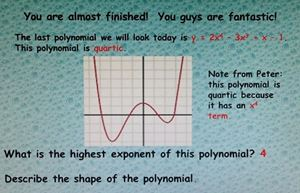In this lesson, students are to identify and define five types of polynomial functions and their graphs. The prefix “poly” means many and the suffix “nomial” means terms. This vocabulary is important as students move forward throughout the remainder of the course.

#### 57 Lesson 2: Adding and Subtracting Polynomials 09:44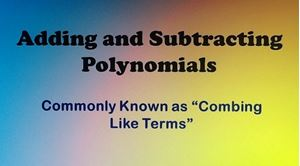In previous lessons, students have identified like terms and combined like terms by adding. This lesson is a continuation of combining like terms. Students find the expressions are more challenging. Remember, to change the subtraction signs to “plus negative” to make the problems easier to simplify.

#### 59 Lesson 3: Multiplying Polynomials Using the Punnett Square 12:10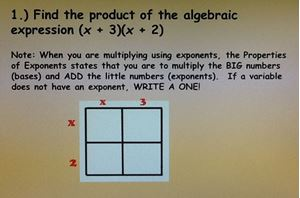In this lesson, students use biology concept of a Punnett square find the product two binomials, and the product of a binomial and a trinomial. This method can be used to find the product of most binomials and trinomials. The “Magic Box” has been of my students’ favorite math lessons!

#### 61 Lesson 1: Bubbly Bria and Factoring the GCF 19:29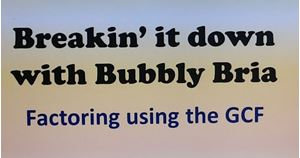In this lesson, students use the concept prime factorization when “breaking down” polynomials. The objective is to find what each term has in common, divide it out of each term, and write the simplified expression.

#### 63 Lesson 2: Factoring Trinomials Using the Tic-Tac-Toe Method 10:45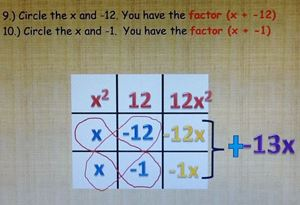In this lesson, students are introduced to theTic-Tac-Toe Method.  This is easiest and most effective way I have found for factoring trinomials. This method works for most general forms of trinomials. Students really enjoy factoring after learning the Tic-Tac-Toe Method!

#### 65 Lesson 3: Factoring a Sum and Difference of Two Cubes 07:42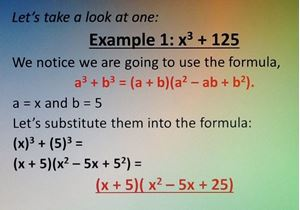In this lesson, students are given a formula for the sum of two cubes and for the difference of two cubes. Students find the values for “a” and “b”, substitute into the formula and then simplify.

#### 67 Lesson 4: Dividing Polynomials 13:34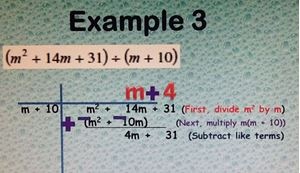This is the last lesson in the course. In this lesson, students divide polynomials using the Laws of Exponents and long division. Students use many of the skills learned throughout the course when dividing polynomials. It is a great way to sum up an algebra course.

#### 68 Dividing Polynomials Independent Practice 19:05

Course Description

This algebra course is specially customized with a solid foundation on number sense and fundamental skills in graphing, systems of equations and inequalities and polynomials. We apply the operations and properties of numbers to solve linear equations and inequalities. We utilize the knowledge of numbers to write, solve and graph systems of linear equations and inequalities. Furthermore, we apply the properties of real numbers to write and to simply polynomial expressions using uniquely designed methods that are both fun and easy.

Goals

• Establish a solid foundation in number sense
• Understand and apply properties of real numbers
• Solve and graph linear equations and linear inequalities
• Solve systems of equations and inequalities
• Apply operations to polynomials
• Use logic and reasoning to help students with proofs in geometry
• Use skills in algebra and apply them to future mathematics courses

Target Audience

This course is designed for students who have completed pre-algebra. The first two modules are devoted to building a solid foundation in number sense and properties of numbers before introducing the concept of a variable. The lessons in this course are designed for students to understand and master each algebra topic so he or she is fully equipped to move on to the next mathematics course with confidence.

Algebra Curriculum

Module 1

Lesson 1: Classification of Sets of Real Numbers

In this lesson, students learn the system that governs and classifies. Numbers are not random, but follow rules and follow into six subsets of numbers in the real number system. Students will learn the six subsets of numbers. When given numbers, students will be able to determine which categories the numbers belong.

Lesson 2: Operations and Properties of Real Numbers

In this lesson, students learn how the four basic operations of numbers (addition, subtraction, multiplication and division) are applied to the properties of numbers. These properties are essential as we learn to simplify expressions and solve equations. In addition, vocabulary is necessary to a successful learning experience.

Lesson 3: Arithmetic Properties of Integers

In this lesson, students learn the rules that govern integers. In lesson 2, we learned what integers are. In this lesson, we apply the rules of integers for addition, subtraction, multiplication and division. It is important to master these rules because we need them when we start solving linear equations and inequalities.

Lesson 4: Arithmetic Properties of Set Notation

In this lesson, we learn how to represent a set of number using mathematical language. There are a lot of symbols in math because if we did not use symbols and short hand, we would more time writing than doing math. The challenging part is to identify the symbol and know it means. I am confident it will be a piece of cake!

Lesson 5: The Closure Property

In this lesson, we learn that when you add two real numbers, we get a real number every time! We know this is always true. However, every operation does not produce a number that belongs to its category. We will look at the four operations and determine which operations are closed and which operations are not closed.

Lesson 6: True and False Assertions

Students are asked to determine if there enough proof for the statement to be TRUE. If not, we have to apply the properties and rules we have learned apply them.  Our goal is to prove and show the validity of the statement. This lesson helps us think logically and give reasonable evidence for our answers.

Module 2

Lesson 1: Can You Tell Me the Order?

In module 1, we discussed the laws and properties that govern the order and the ranks of sets of numbers in the real number system. In this lesson 1, we are going to apply the properties of numbers. In this lesson is called, “Can You Tell Me the Order?” because students apply the rules and the operations of numbers to determine the correct answer. Students discover the order of the operations matter when working out the problem.  You may find a four-function is helpful for the independent practice.

Lesson 2: Understanding and Using Operations: Opposites and Reciprocals

In Module 1, we learned about the additive inverse and the multiplicative inverse of a number. It will be helpful to refer back to the properties of numbers in module 1 because these properties are used in module 2. Students use these properties when talking about opposites and reciprocals. We discover that the additive inverse is called the opposite of a numbers; the multiplicative inverse is called the reciprocal of a number. These four terms are the essence of this lesson. However, the mathematics behind these words is a piece of cake!

Lesson 3: Understanding and Using Operations: Roots and Fractional Powers

In this lesson, we began by looking at the properties of square roots and cube roots. This concept helps us better understand fractional powers. It is important to take notes and pay close attention to the vocabulary used throughout the lesson. Don’t worry! Fractional powers are not as frightening as they may sound. We find the product of the root and whole number powers to find the answer. I have provided a list of square roots and cube roots that you will find very helpful as we proceed through this lesson.

Lesson 4: Laws of Exponents

In this lesson, we look at the rules and properties that govern exponents. An exponent is short hand for multiplication. It is very important for students to know and be able to apply all the laws of exponents. We find that there is more than one law that is applied in a single problem. We also investigate the rules for exponents involving multiplication and division. In addition, we learn how the reciprocal of a number is in important when simplifying exponents.

Lesson 5: Variables and Like Terms

In this lesson, students are introduced to the variable and the vocabulary that surrounds it. We investigate what a term is, and determine if two terms are like terms or unlike terms. This is important because this lesson is all about simplifying expressions and adding like terms together. This skill is necessary throughout the remainder of the course. It is essential for students to be proficient in simplify and combining like terms.

In this video, I provide a detailed explanation for all the problems in the independent practice. Students are to become confident in changing the sign, applying the Distributive Property and combining like terms.

Lesson 6: “Can You Accept That to be True” Deductive and Inductive Reasoning

In this lesson, students are introduced to two types of logical reasoning for problem solving: deductive reasoning and inductive reasoning. Mathematics primarily uses deductive reasoning for its method of finding the solution; there is one correct answer. However, when we are looking for a pattern, then we may use inductive reasoning. Students may find that inductive reasoning is mostly the logical means of reasoning in science.

Lesson 7: Under What Conditions is That Statement True? Hypothesis and Conclusion

In this lesson, students discover how to determine if a statement is true or false from the given information. Next, students use deductive reasoning to identify the hypothesis and conclusion of a statement. Furthermore, we are going to write a conditional statement when we are given the hypothesis and conclusion. We learn that a counterexample is an example that shows a conditional statement is false.

Module 3

Lesson 1: Simplifying Expressions

This lesson is a continuation of Lesson 5 from Module 2, “Variables and Like Terms.” In lesson 1 of Module 3, we are going to simplify more complex expressions that are more commonly known as algebraic expressions. Students are to apply the Laws of Exponents, the Distributive and Property and combining like terms to successfully simplify each expression. This skill is very important when we solve linear equations in the next lesson.

Lesson 2: Ecstatic Ellen and Equations: Solving Linear Equations

In this lesson, Ecstatic Ellen is your tour guide. She is excited about solving equations! She knows a fun and an easy to way to solve the most challenging equations using the Barnes Method. Students become confident and excited about being able to solve equations! Let’s get started!

Lesson 3: Ecstatic Ellen and Inequalities: Solving and Graphing Linear Inequalities

In this lesson, students use the same skills needed to solve liner equation. However, students are introduced to the four inequality symbols, determine whether circle on the number line is opened or closed, and determine whether the inequality which direction to shade on the number line. You may find a color pencil to be helpful in this lesson.

Lesson 4: Problem Solving Involving Equations: Using the GUESS Method.

There is no need to fear word problems. In this lesson, students are introduced to easy and fun way to solve word problems. It is called the GUESS Method. Students determine if the answer they calculate is reasonable. Students learn what GUESS stands for and how it use the method to confidently set up and solve word problems with ease!

Lesson 5: Problem Solving Involving Inequalities: Using the GUESS Method

In this lesson, students use the GUESS Method to solve word problems. It is important for students to make sure their answer makes sense in the context of the problem. Students have may have to round or estimate when giving their answers.

Lesson 6: Solving and Describing Absolute Value Equations

In this lesson, students learn what absolute value means and it applies when solving absolute value equations. Students are expected to be able to write the two equations, solve the two equations, and describe the answers in words. Written communication is helpful in conceptualizing the answer.

Lesson 7: Solving and Describing Absolute Value Inequalities

In this lesson, students apply their knowledge of graphing inequalities. Students are expected to able to write the two inequalities, solve and graph the inequalities, and describe the solution in words. Written communication is helpful in conceptualizing the answer.

Module 4

Lesson 1: Graphing Linear Equations

In this lesson, graph paper is provided. Students need a ruler. Students are given a set of x-values they use to substitute into the equation. The y-value is the answer needed to form the ordered pairs. The ordered pairs are then plotted on the coordinate plane form the linear equation.

Lesson 2: Graphing Linear Inequalities.

In this lesson, graph paper is provided. Students need a ruler, a highlighter and colored pencils. They are some similarities in graphing equations and graphing inequalities. However, when graphing inequalities, students must determine which area on the graph is the solution set.

Lesson 3: Help Me Find My Equation! Point-Slope Formula

In this lesson, I write a story that involves three friends: Slope and his two friends X and Y. They are sad because they cannot find their equation. Slope, X and Y need your help to find the equation using the Point-Slope Formula. This lesson is a lot of fun!

In this video, I provide a detailed explanation of how to help Slope and his friends find their equation using the Point-Slope Formula. You find they are very grateful for your help and cooperation!

Lesson 4: What’s the Slope? Solving for Y and Identifying the Slope of an Equation

In this lesson, students learn how to write a two-variable equation in slope-intercept form. Students used the Barnes Method for using x, and use it to solve the equations for y. Now that the equations are slope-intercept form, students identify the slope, y-intercept, and determine whether the line increases, decreases, constant or undefined.

Module 5

Lesson 1: Systems of Linear Equations. Part 1

There are two parts to solving systems of linear equations. In part 1 of this lesson, students solve systems of linear equations by graphing and substitution. The point of intersection is called the    solution of system of linear equations when we are graphing. When using substitution, you solve the first equation for y, and substitute the FIRST into the SECOND equation. Graph paper is        provided.

Lesson 2: Systems of Linear Equations. Part 2

In part of this lesson, students solve systems of linear equations by using elimination. In the Elimination Method, you have to eliminate or cancel out a variable. We want one of the terms to have the same number so we can ADD the two equations together.

Lesson 3: What’s the Solution Set? Graphing Systems of Linear Inequalities.

In this lesson, students generate a table of values to plot on the coordinate plane. Based on the inequality symbol, students determine whether the line that is drawn is solid or dashed; students also determine whether they must shade below or above the line. The area in which the two inequalities intersect is the solution set. Students need a ruler, a highlighter and colored pencils.

Module 6

Lesson 1: Polynomial Basics with Powerful Peter.

In this lesson, students are to identify and define five types of polynomial functions and their graphs. The prefix “poly” means many and the suffix “nomial” means terms. This vocabulary is important as students move forward throughout the remainder of the course.

Lesson 2: Adding and Subtracting Polynomials

In previous lessons, students have identified and like terms and combined like terms by adding. This lesson is a continuation of combining like terms. Students find the expressions are more challenging. It is important for students to change the problems to addition problems in order to make the problems easier to simplify.

Lesson 3: Multiplying Polynomials Using the Punnett Square

In this lesson, students use biology concept of a Punnett square find the product two binomials, and the product of a binomial and a trinomial. This method can be used to find the product of most binomials and trinomials. The “Magic Box” has been of my students’ favorite activities to do.

Module 7

Lesson 1: Bubbly Bria and Factoring the GCF

In this lesson, students use the concept prime factorization when “breaking down” polynomials. The objective is to find what each term has in common, divide it out of each term, and write the simplified expression.

In this video, I provide a detailed explanation of how to find the greatest common factor, how to find divide it out of the expression, and how to write the simplified expression.

Lesson 2: Factoring Trinomials Using the Tic-Tac-Toe Method

The Tic-Tac-Toe Method is easiest and most effective way I have found for factoring trinomials. This method works for most general forms of trinomials. Students really enjoy factoring after learning the Tic-Tac-Toe Method!

In this video, I provide a detailed explanation of how to factor trinomials using the Tic-Tac-Toe Method. Be prepared to be amazed!

Lesson 3: Factoring a Sum and Difference of Two Cubes

In this lesson, students are given a formula for the sum of two cubes and for the difference of two cubes. Students find the values for “a” and “b”, substitute into the formula and then simplify.

In this video, I provide a detailed explanation of how to use the formulas for a sum and a difference of two cubes. This is a straightforward lesson that uses formulas, substitution, and simplifying expressions.

Lesson 4: Dividing Polynomials

This is the last lesson in the course. In this lesson, students divide polynomials using the Laws of Exponents and long division. Students use many of the skills learned throughout the course when dividing polynomials. It is a great way to sum up an algebra course.

Course Includes:

• 15 hours of video
• 34 Video-lessons
• 33 Independent Practice Videos
• 7 Module Tests
• 8 Power points
• 20 Lesson handouts
• End of Course Exam with Answers

Course Topics

• Using Arithmetic Properties: Basics
• Using Arithmetic Properties: Operations and Properties
• Using Arithmetic Properties: Integers
• Using Arithmetic Properties: Set Notation
• Using Arithmetic Properties: Closure Properties
• True and False Assertions
• Understanding and Using Operations: Basics
• Understanding and Using Operations: Opposites and Reciprocals
• Understanding and Using Operations: Roots and Fractional Powers
• Understanding and Using Operations: Rules of Exponents
• Understanding and Using Operations: Variables and Like Terms
• Inductive and Deductive Reasoning
• Hypothesis and Conclusion
• Simplifying Expressions
• Solving Linear Equations Using the Barnes Method
• Solving Multistep Linear Inequalities on a Number Line
• Solving Problem Situations Involving Equation Using the GUESS Method
• Solving Problem Situations Involving Inequalities Using the GUESS Method
• Solving Equations with Absolute Value
• Solving Inequalities with Absolute Value
• Linear Equation Graphs
• Linear Inequality Graphs
• Point-Slope Formula
• Related Slopes: Solving for Y and Identifying the Slope and Y-Intercept
• Systems of Two Linear Equations in Two Variables
• Part One: Graphing and Substitution
• Part Two: Elimination Method
• Systems of Two Linear Inequalities in Two Variables
• Working with Polynomials: Basics
• Working with Polynomials: Adding and Subtracting
• Working With Polynomials: Multiplication Using the Punnett Square
• Factoring Polynomials: Using the GCF
• Factoring Polynomials: Sum and Difference of Two Cubes
• Dividing Polynomials using Long Division

• Teacher: Canaa
• Areas of expertise: Algebra 1
• Education: Master of Arts, Major: Mathematics - University of Central Arkansas, Conway, AR (2005) Bachelor of Science in Education, Major: Mathematics - Arkansas State University, Jonesboro, AR (2001) Secondary Education Certification
• Interests:
• Skills: EXPERIENCE IN: Researching information Curriculum writing Use of Technology in Classroom Motivating Students and Teachers Educational Consulting and Services Multi-ethnic populations Current trends in instruction and teaching strategies Communication and interpersonal skills Planning Events Professional Development Instructional Leadership
• Associations:
• Issues I care about: Teacher Effectiveness Instructional Delivery Teaching Strategies

Every child is capable to mastering mathematics. In order for mastery to be achieved, students must be provided with meaningful learning experiences; an opportunity to conceptualize and internalize the mathematics, and to experience a progression through mathematics that is related to their prior knowledge.

#### Module 1 Test

For each of the following problems, choose the answer the best represents the property of real numbers.

Properties of Real Numbers

#### Module 2 Test

For each of the following problems, choose the answer that best represents the problem situation.

Operations of Real Numbers

#### Module 3 Test

For each of the following problems, choose the answer that best represents the problem situation.

For each of the following problems, choose the answer that best represents the problem situation.

#### Module 4 Test

For each of the following problems, choose the answer that best represents the problem situation.

Graphing Lines and Finding the Slope

#### Module 5

For each of the following problems, choose the answer that best represents the problem situation.

Systems of Equations and Inequalities

#### Module 6 Test

For each of the following problems, choose the answer that best represents the problem situation.

Polynomial Basics

#### Module 7 Test

For each of the following problems, choose the answer that best represents the problem situation.